Ex 9.3

Chapter 9 Class 11 Sequences and Series (Term 1)
Serial order wise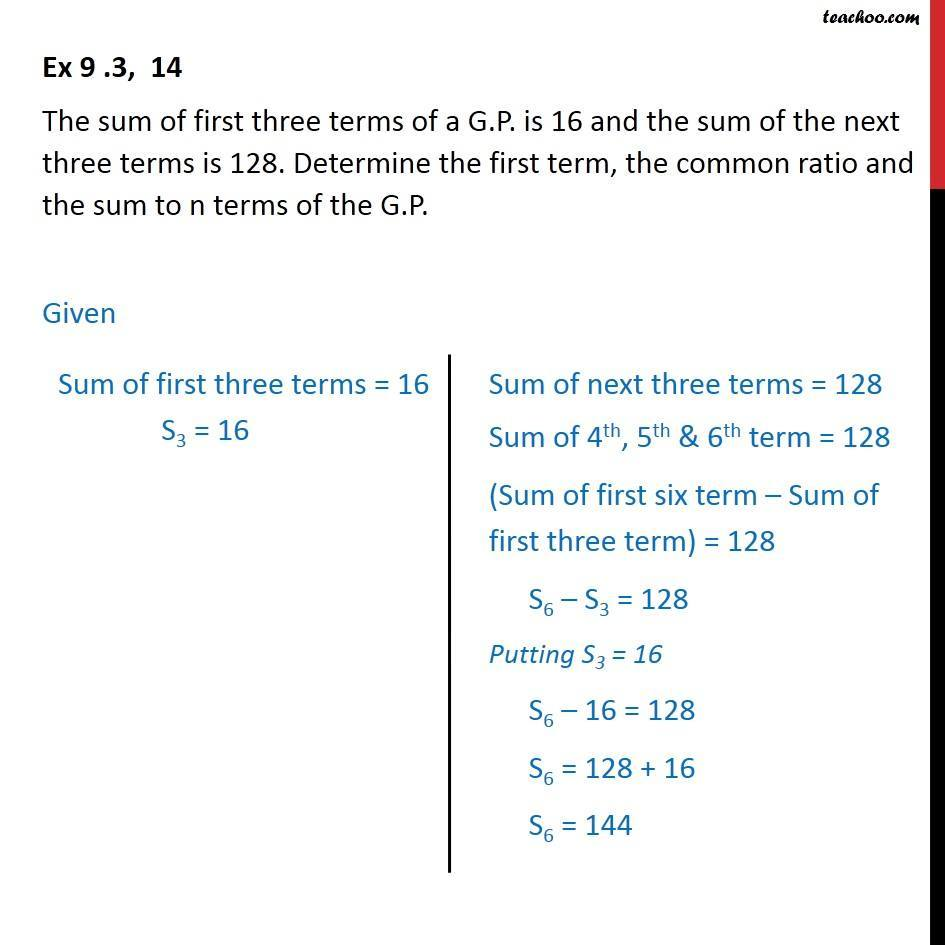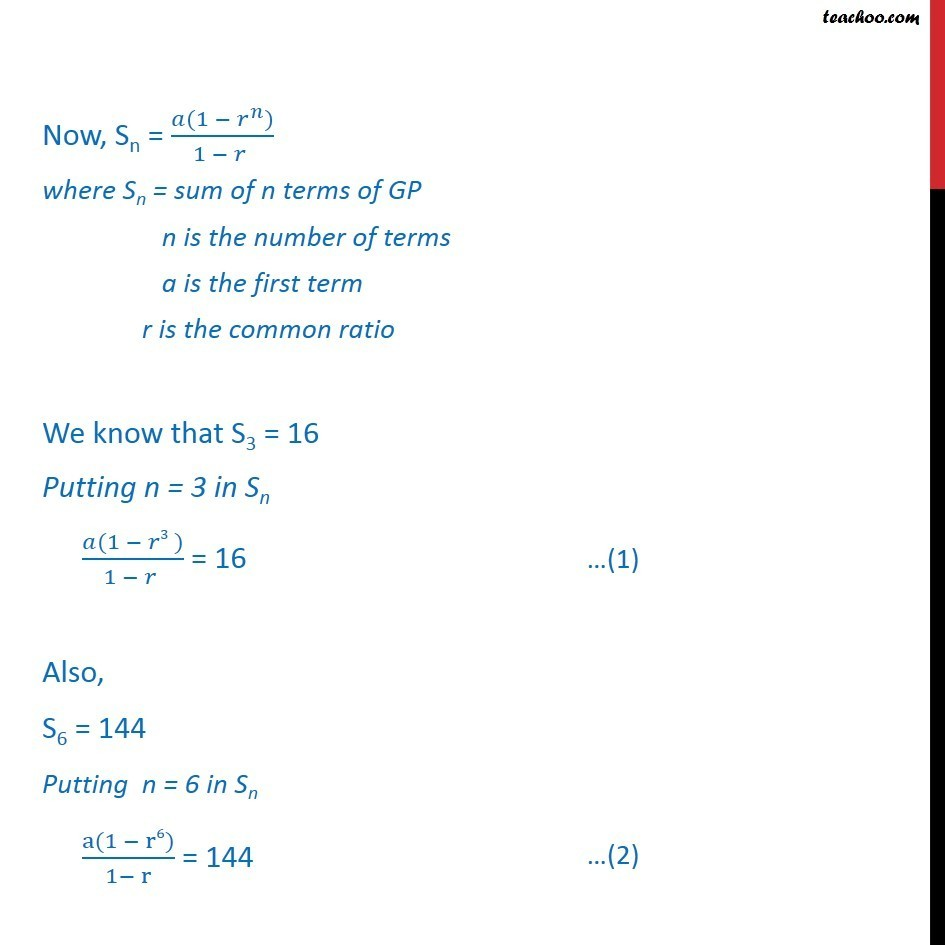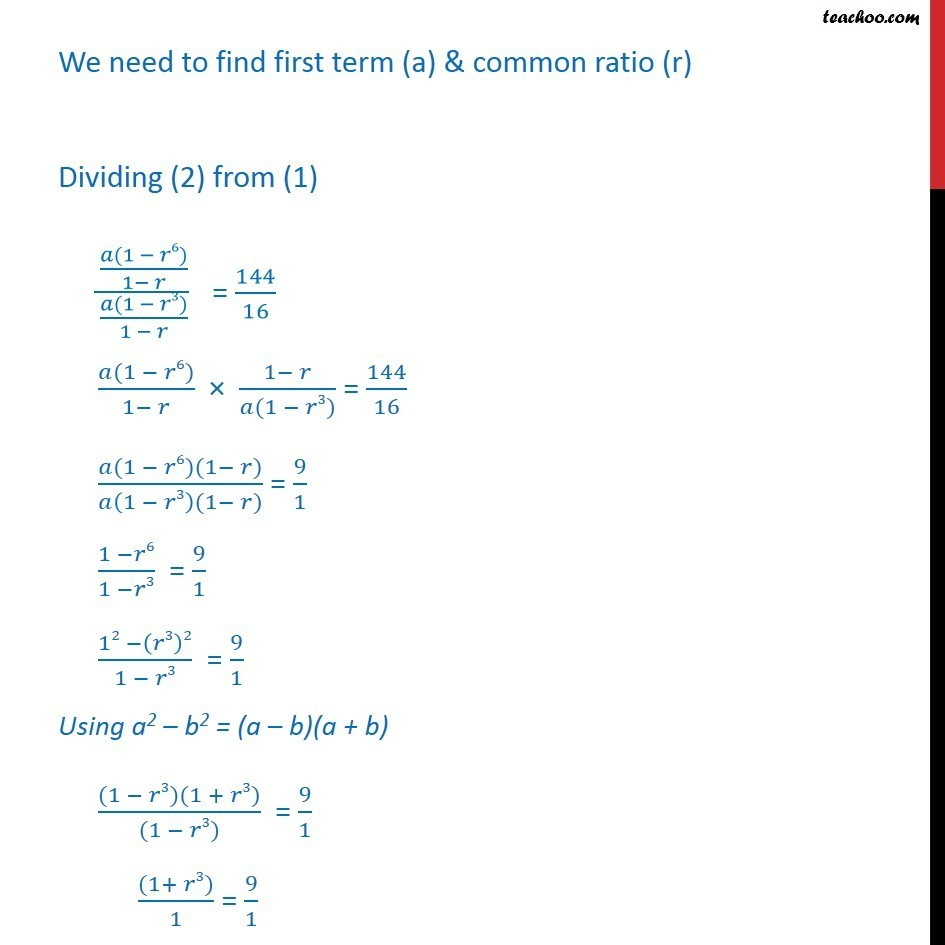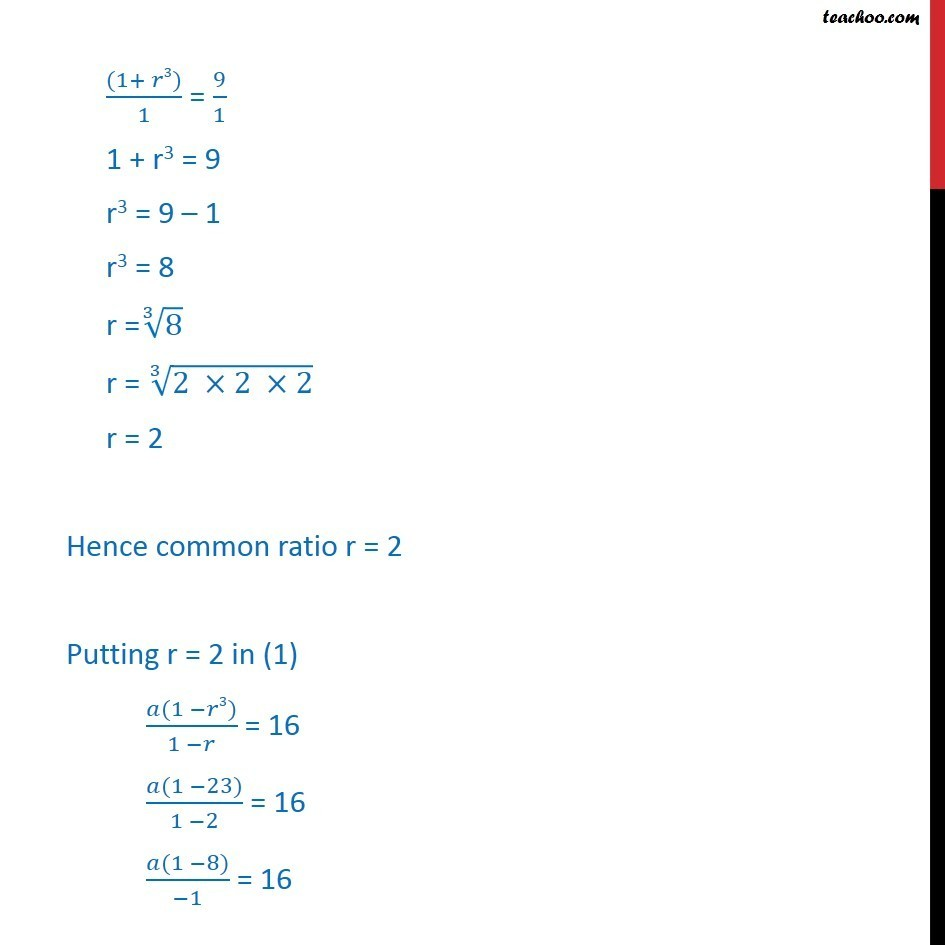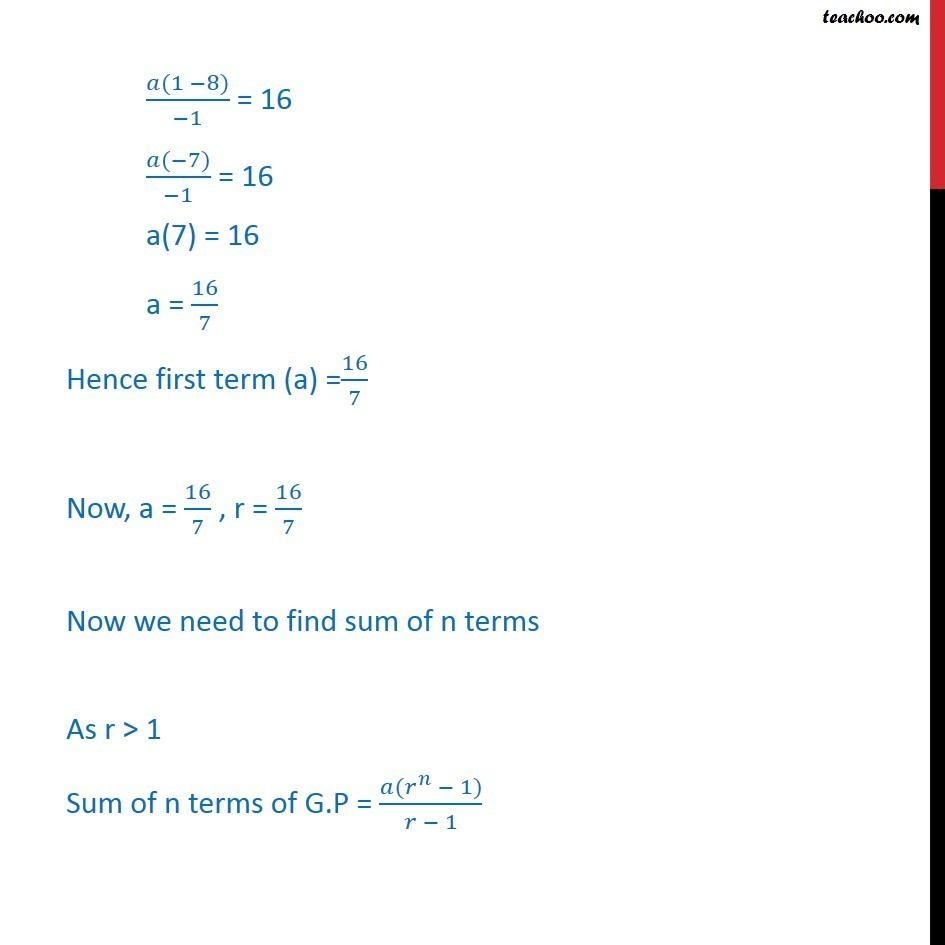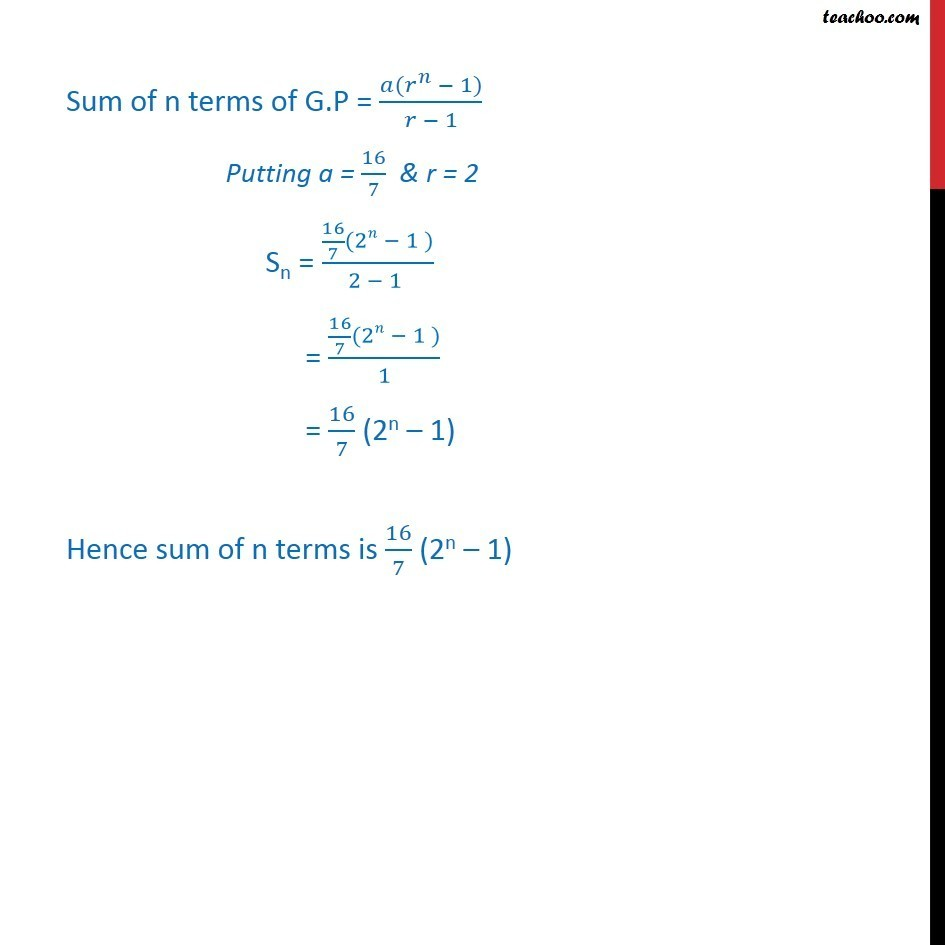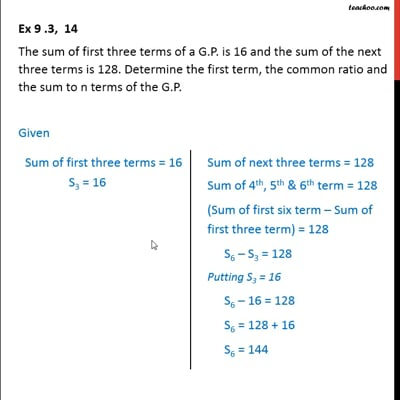This video is only available for Teachoo black users

### Transcript

Ex 9 .3, 14 The sum of first three terms of a G.P. is 16 and the sum of the next three terms is 128. Determine the first term, the common ratio and the sum to n terms of the G.P. Given Now, Sn = (𝑎(1 − 𝑟^𝑛))/(1 − 𝑟 ) where Sn = sum of n terms of GP n is the number of terms a is the first term r is the common ratio We know that S3 = 16 Putting n = 3 in Sn (𝑎(1 − 𝑟3 ))/(1 − 𝑟 ) = 16 Also, S6 = 144 Putting n = 6 in Sn (a(1 − r6))/(1− r) = 144 We need to find first term (a) & common ratio (r) Dividing (2) from (1) ((𝑎(1 − 𝑟6))/(1− 𝑟))/((𝑎(1 − 𝑟3))/(1 − 𝑟)) = 144/16 (𝑎(1 − 𝑟6))/(1− 𝑟) × (1− 𝑟)/(𝑎(1 − 𝑟3)) = 144/16 (𝑎(1 − 𝑟6)(1− 𝑟))/(𝑎(1 − 𝑟3)(1− 𝑟)) = 9/1 (1 −𝑟6)/(1 −𝑟3) = 9/1 (12 −(𝑟3)2)/(1 − 𝑟3) = 9/1 Using a2 – b2 = (a – b)(a + b) ((1 − 𝑟3)(1 + 𝑟3))/((1 − 𝑟3)) = 9/1 ((1+ 𝑟3))/1 = 9/1 ((1+ 𝑟3))/1 = 9/1 1 + r3 = 9 r3 = 9 – 1 r3 = 8 r =∛8 r = ∛(2 ×2 ×2) r = 2 Hence common ratio r = 2 Putting r = 2 in (1) (𝑎(1 −𝑟3))/(1 −𝑟) = 16 (𝑎(1 −23))/(1 −2) = 16 (𝑎(1 −8))/(−1) = 16 (𝑎(1 −8))/(−1) = 16 (𝑎(−7))/(−1) = 16 a(7) = 16 a = 16/7 Hence first term (a) =16/7 Now, a = 16/7 , r = 16/7 Now we need to find sum of n terms As r > 1 Sum of n terms of G.P = (𝑎(𝑟^𝑛 − 1))/(𝑟 − 1) Sum of n terms of G.P = (𝑎(𝑟^𝑛 − 1))/(𝑟 − 1) Putting a = 16/7 & r = 2 Sn = (16/7(2𝑛 − 1 ))/(2 − 1 ) = (16/7(2𝑛 − 1 ))/1 = 16/7 (2n – 1) Hence sum of n terms is 16/7 (2n – 1)